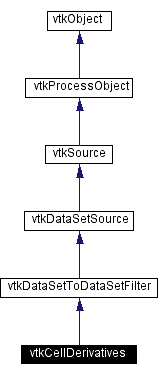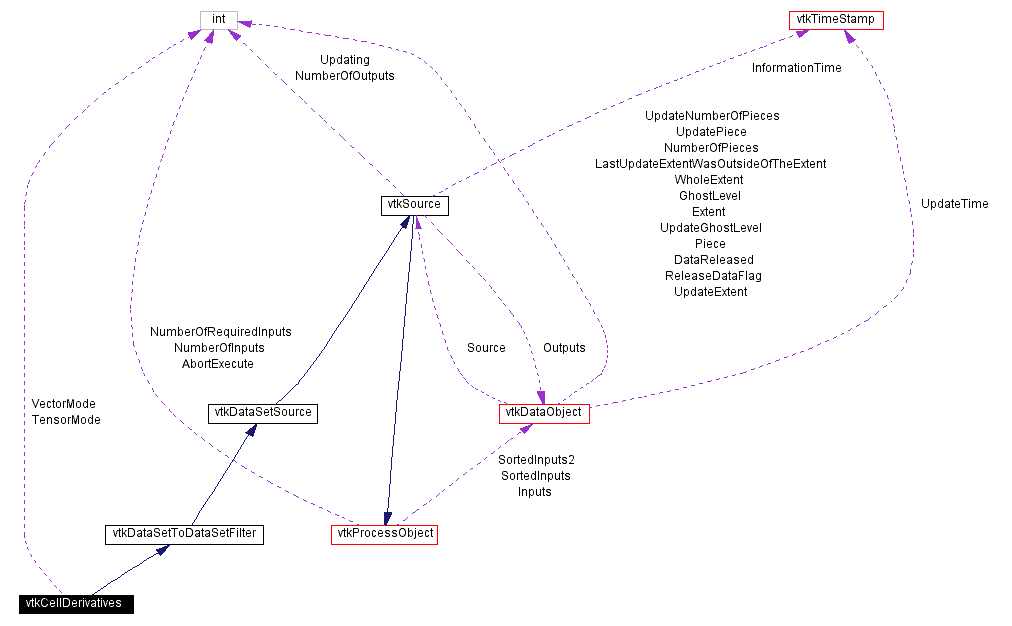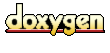Main Page   Class Hierarchy   Alphabetical List   Compound List   File List   Compound Members   File Members   Related Pages

# vtkCellDerivatives Class Reference

compute derivatives of scalars and vectors. More...

`#include <vtkCellDerivatives.h>`

Inheritance diagram for vtkCellDerivatives:[legend]
Collaboration diagram for vtkCellDerivatives:[legend]
List of all members.

## Public Methods

virtual const char * GetClassName ()
virtual int IsA (const char *type)
void PrintSelf (ostream &os, vtkIndent indent)
virtual void SetVectorMode (int)
virtual int GetVectorMode ()
void SetVectorModeToPassVectors ()
void SetVectorModeToComputeVorticity ()
const char * GetVectorModeAsString ()
virtual void SetTensorMode (int)
virtual int GetTensorMode ()
void SetTensorModeToPassTensors ()
void SetTensorModeToComputeStrain ()
const char * GetTensorModeAsString ()

## Static Public Methods

int IsTypeOf (const char *type)
vtkCellDerivatives * SafeDownCast (vtkObject *o)
vtkCellDerivatives * New ()

## Protected Methods

vtkCellDerivatives ()
~vtkCellDerivatives ()
vtkCellDerivatives (const vtkCellDerivatives &)
void operator= (const vtkCellDerivatives &)
void Execute ()

int VectorMode
int TensorMode

## Detailed Description

compute derivatives of scalars and vectors.

Date:
2000/12/10 20:08:22
Revision:
1.12

vtkCellDerivatives is a filter that computes derivatives of scalars and vectors at the center of cells. You can choose to generate different output including the scalar gradient (a vector), computed tensor vorticity (a vector), gradient of input vectors (a tensor), and strain matrix of the input vectors (a tensor); or you may choose to pass data through to the output.

Note that it is assumed that on input scalars and vector point data is available, which are then used to generate cell vectors and tensors. (The interpolation functions of the cells are used to compute the derivatives which is why point data is required.)

Warning:
The computed derivatives are cell attribute data; you can convert them to point attribute data by using the vtkCellDataToPointData filter. Note that, due to the interpolation function used (obtained using 1/r**2 normalized sum), the derivatives calculated for polygons with more than 4 vertices are inaccurate in most cases.
Warning:
The point data is passed through the filter to the output.
vtkVectorNorm
Examples:
vtkCellDerivatives (examples)

Definition at line 87 of file vtkCellDerivatives.h.

## Constructor & Destructor Documentation

 vtkCellDerivatives::vtkCellDerivatives ( ) ` [protected]`

 vtkCellDerivatives::~vtkCellDerivatives ( ) ` [inline, protected]`
 Definition at line 128 of file vtkCellDerivatives.h.

 vtkCellDerivatives::vtkCellDerivatives ( const vtkCellDerivatives & ) ` [inline, protected]`
 Definition at line 129 of file vtkCellDerivatives.h.

## Member Function Documentation

 virtual const char* vtkCellDerivatives::GetClassName ( ) ` [virtual]`
 Return the class name as a string. This method is defined in all subclasses of vtkObject with the vtkTypeMacro found in vtkSetGet.h. Reimplemented from vtkDataSetToDataSetFilter.

 int vtkCellDerivatives::IsTypeOf ( const char * type ) ` [static]`
 Return 1 if this class type is the same type of (or a subclass of) the named class. Returns 0 otherwise. This method works in combination with vtkTypeMacro found in vtkSetGet.h. Reimplemented from vtkDataSetToDataSetFilter.

 virtual int vtkCellDerivatives::IsA ( const char * type ) ` [virtual]`
 Return 1 if this class is the same type of (or a subclass of) the named class. Returns 0 otherwise. This method works in combination with vtkTypeMacro found in vtkSetGet.h. Reimplemented from vtkDataSetToDataSetFilter.

 vtkCellDerivatives* vtkCellDerivatives::SafeDownCast ( vtkObject * o ) ` [static]`
 Will cast the supplied object to vtkObject* is this is a safe operation (i.e., a safe downcast); otherwise NULL is returned. This method is defined in all subclasses of vtkObject with the vtkTypeMacro found in vtkSetGet.h. Reimplemented from vtkDataSetToDataSetFilter.

 void vtkCellDerivatives::PrintSelf ( ostream & os, vtkIndent indent ) ` [virtual]`
 Methods invoked by print to print information about the object including superclasses. Typically not called by the user (use Print() instead) but used in the hierarchical print process to combine the output of several classes. Reimplemented from vtkSource.

 vtkCellDerivatives* vtkCellDerivatives::New ( ) ` [static]`
 Construct to compute the gradient of the scalars and vectors. Reimplemented from vtkDataSetSource.

 virtual void vtkCellDerivatives::SetVectorMode ( int ) ` [virtual]`
 Control how the filter works to generate vector cell data. You can choose to pass the input cell vectors, compute the gradient of the input scalars, or extract the vorticity of the computed vector gradient tensor. By default (VectorModeToComputeGradient), the filter will take the gradient of the input scalar data.

 virtual int vtkCellDerivatives::GetVectorMode ( ) ` [virtual]`

 void vtkCellDerivatives::SetVectorModeToPassVectors ( ) ` [inline]`
 Definition at line 103 of file vtkCellDerivatives.h.

 void vtkCellDerivatives::SetVectorModeToComputeGradient ( ) ` [inline]`
 Definition at line 105 of file vtkCellDerivatives.h.

 void vtkCellDerivatives::SetVectorModeToComputeVorticity ( ) ` [inline]`
 Definition at line 107 of file vtkCellDerivatives.h.

 const char* vtkCellDerivatives::GetVectorModeAsString ( )

 virtual void vtkCellDerivatives::SetTensorMode ( int ) ` [virtual]`
 Control how the filter works to generate tensor cell data. You can choose to pass the input cell tensors, compute the gradient of the input vectors, or compute the strain tensor of the vector gradient tensor. By default (TensorModeToComputeGradient), the filter will take the gradient of the vector data to construct a tensor.

 virtual int vtkCellDerivatives::GetTensorMode ( ) ` [virtual]`

 void vtkCellDerivatives::SetTensorModeToPassTensors ( ) ` [inline]`
 Definition at line 118 of file vtkCellDerivatives.h.

 void vtkCellDerivatives::SetTensorModeToComputeGradient ( ) ` [inline]`
 Definition at line 120 of file vtkCellDerivatives.h.

 void vtkCellDerivatives::SetTensorModeToComputeStrain ( ) ` [inline]`
 Definition at line 122 of file vtkCellDerivatives.h.

 const char* vtkCellDerivatives::GetTensorModeAsString ( )

 void vtkCellDerivatives::operator= ( const vtkCellDerivatives & ) ` [inline, protected]`
 Definition at line 130 of file vtkCellDerivatives.h.

 void vtkCellDerivatives::Execute ( ) ` [protected, virtual]`
 Reimplemented from vtkSource.

## Member Data Documentation

 int vtkCellDerivatives::VectorMode` [protected]`
 Definition at line 133 of file vtkCellDerivatives.h.

 int vtkCellDerivatives::TensorMode` [protected]`
 Definition at line 134 of file vtkCellDerivatives.h.

The documentation for this class was generated from the following file:

Generated on Wed Nov 21 12:46:42 2001 for VTK by1.2.11.1 written by Dimitri van Heesch, © 1997-2001